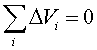Kirchhoff's laws

Most of the circuit problems we encounter can be solved by repeatedly applying the rules for adding resistors in series or parallel, until the problem has been reduced to one of a battery connected to a single resistor.

But to solve more complex circuit problems, such as those with more than one battery, it is sometimes necessary instead to write equations based on Kirchhoff's Laws, which are formal mathematical statements of two physical facts that you already know:

• Kirchhoff's law #1   states that the voltage changes around a closed path in a circuit add up to zero, where the voltage change DV = emf in going through a battery from - terminal to + terminal is considered to be positive, and the voltage change DV = I R in going through a resistor in the assumed direction of the current I is considered to be negative.,

• Kirchhoff's law #2   states that the sum of the currents entering any node (i.e., any junction of wires) equals the sum of the currents leaving that node.
• The first law just restates what you already know about electrical potential: every point in a circuit has a unique value of the potential, so travelling around the circuit by any path must bring you back to the potential you started from. Using the analogy to elevation, if you hike from any starting point in the mountains and wander around by any choice of paths but finish at your original starting point, the sum of the elevation changes along your path will add up to zero.

The second law just restates the fact that electric charge is conserved: electrons or protons are not being created or destroyed in the node (or if they are, anti-particles with the opposite charge are being created or destroyed along with them) so in any given time interval, the charge that enters is equal to the charge the leaves. The node is assumed to have negligible capacitance, so charge cannot just build up there. For example, at a point where three wires are connected as in the diagram below, charge conservation requires that i1 = i2 + i3.